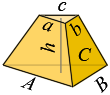### Volume of Triangular FrustumDimensions:

Bottom base

A = , B = , C =

Top base

a = , b = B·aA , c = C·aA

Height - h =

Bottom base

Semiperimeter

p = 0.5·(A + B + C)

Area (Heron equation)

A1 =  p·(pA)·(pB)·(pC)

Top base

Semiperimeter

p = 0.5·(a + b + c)

Area (Heron equation)

A2 =  p·(pa)·(pb)·(pc)

Volume

V = h3·(A1 +  A1·A2 + A2)

The sum of each two sides must be greater than the third.